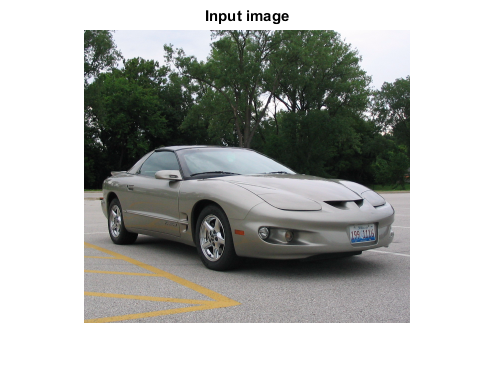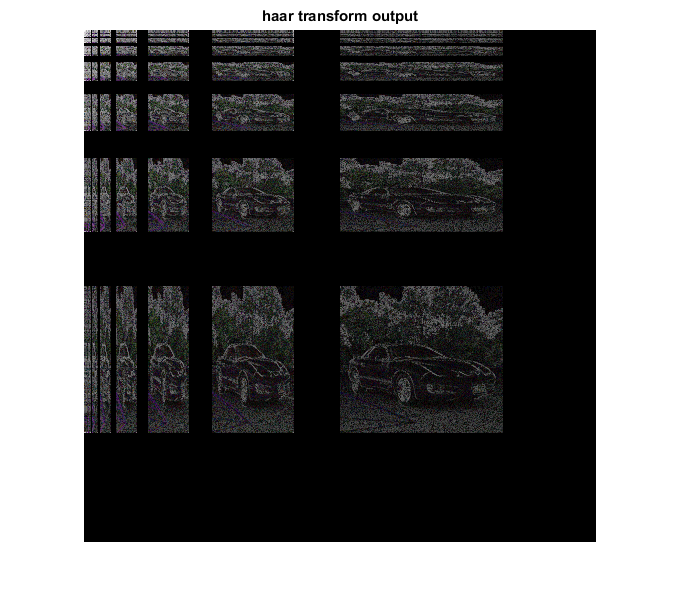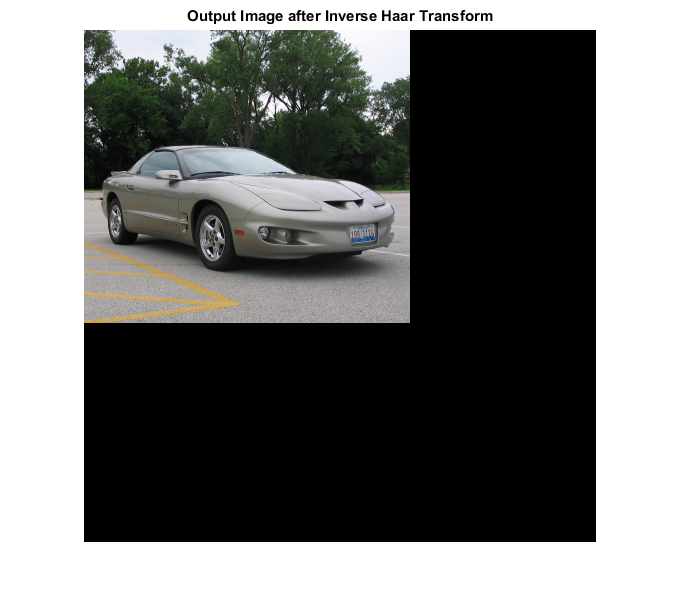# IHAAR_CVIP

ihaar_cvip() - performs inverse Haar transform.

## SYNTAX

`img = ihaar_cvip( input_spect, block_size )`

Input Parameters include:

• input_spect - The image haar spectrum. This spectrum must be obtained by haar_cvip().
• block_size - Block_size of the inverse transform should be same as the block_size used for the forward Haar transform.

Output Parameter include :

• img - The inverse haar transform of the input spectrum.

## DESCRIPTION

This function computes the inverse haar transform of the input image. The argument block_size should match the same value given for the forward Haar transform.

## REFERENCE

1. Scott E Umbaugh. DIGITAL IMAGE PROCESSING AND ANALYSIS: Applications with MATLAB and CVIPtools, 3rd Edition.

## EXAMPLE

```% Read Image

% Calling haar function

spect = haar_cvip( I,[] );

% use abs to compute the magnitude (handling imaginary) and use log to brighten display

X=log(1+abs(spect));

X = remap_cvip(X);

% Calling inverse haar transform function

Output = ihaar_cvip(spect,[]);

% Display input image

figure;imshow(I);title('Input image');

% Display output images

figure;imshow(X);title('haar transform output')

figure;imshow(hist_stretch_cvip(Output,0,1,0,0));title('Output Image after Inverse Haar Transform');
```